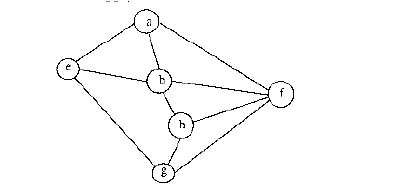Related Articles

# Algorithms | Graph Traversals | Question 12

• Last Updated : 15 Nov, 2018

Consider the following graph,Among the following sequences:

```(I) a b e g h f
(II) a b f e h g
(III) a b f h g e
(IV) a f g h b e  ```

Which are depth first traversals of the above graph?
(A) I, II and IV only
(B) I and IV only
(C) II, III and IV only
(D) I, III and IV only

Explanation: We can check all DFSs for following properties.

```In DFS, if a vertex 'v' is visited
after 'u', then one of the following is true.
1) (u, v) is an edge.
u
/   \
v     w
/     / \
x     y   z

2) 'u' is a leaf node.
w
/   \
x     v
/     / \
u     y   z```

In DFS, after visiting a node, we first recur for all unvisited children. If there are no unvisited children (u is leaf), then control goes back to parent and parent then visits next unvisited children.

Quiz of this Question

My Personal Notes arrow_drop_up